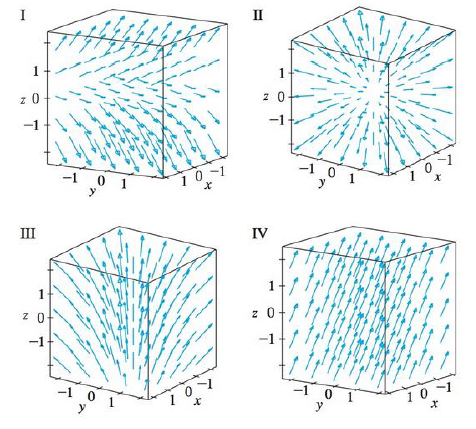Chapter 16.1, Problem 16E

Chapter
Section
Textbook Problem

Match the vector fields F on ℝ3 with the plots labeled I-IV. Give reasons for your choices.16. F(x, y, z) = i + 2 j + z kTo determine

To match: The vector field F(x,y,z)=i+2j+zk with the plots labeled as I-IV.

Explanation

Given data:

F(x,y,z)=i+2j+zk=1,2,z

Formula used:

Consider a three-dimensional vector F=x,y,z .

Write the expression for length of the three dimensional vector.

|F(x,y,z)|=x2+y2+z2 (1)

Find the length of F(x,y,z) using equation (1)

Still sussing out bartleby?

Check out a sample textbook solution.

See a sample solution

The Solution to Your Study Problems

Bartleby provides explanations to thousands of textbook problems written by our experts, many with advanced degrees!

Get Started

In Exercises 1728, use the logarithm identities to obtain the missing quantity.

Finite Mathematics and Applied Calculus (MindTap Course List)

Evaluate the integral. 03(2sinxex)dx

Single Variable Calculus: Early Transcendentals, Volume I

A sample of n = 9 scores has X = 108. What is the sample mean?

Essentials of Statistics for The Behavioral Sciences (MindTap Course List)

53-54 Find f(n)(x). f(x)=2x

Calculus (MindTap Course List)

8273

Applied Calculus for the Managerial, Life, and Social Sciences: A Brief Approach

In problems 23-58, perform the indicated operations and simplify. 37.

Mathematical Applications for the Management, Life, and Social Sciences

Factor: 3y6

Elementary Technical Mathematics

For and

Study Guide for Stewart's Multivariable Calculus, 8th

For y = sin 6x, dydx=. a) cos 6x b) 6 cos x c) cos 6 d) 6 cos 6x

Study Guide for Stewart's Single Variable Calculus: Early Transcendentals, 8th

Graph each rational function. f(x)=x2x2x2+x2

College Algebra (MindTap Course List)